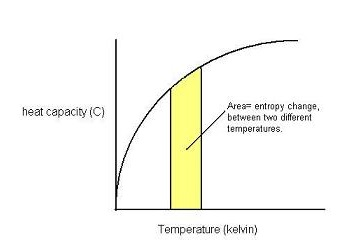# 3rd Law of Thermodynamics

$$\newcommand{\vecs}{\overset { \rightharpoonup} {\mathbf{#1}} }$$ $$\newcommand{\vecd}{\overset{-\!-\!\rightharpoonup}{\vphantom{a}\smash {#1}}}$$$$\newcommand{\id}{\mathrm{id}}$$ $$\newcommand{\Span}{\mathrm{span}}$$ $$\newcommand{\kernel}{\mathrm{null}\,}$$ $$\newcommand{\range}{\mathrm{range}\,}$$ $$\newcommand{\RealPart}{\mathrm{Re}}$$ $$\newcommand{\ImaginaryPart}{\mathrm{Im}}$$ $$\newcommand{\Argument}{\mathrm{Arg}}$$ $$\newcommand{\norm}{\| #1 \|}$$ $$\newcommand{\inner}{\langle #1, #2 \rangle}$$ $$\newcommand{\Span}{\mathrm{span}}$$ $$\newcommand{\id}{\mathrm{id}}$$ $$\newcommand{\Span}{\mathrm{span}}$$ $$\newcommand{\kernel}{\mathrm{null}\,}$$ $$\newcommand{\range}{\mathrm{range}\,}$$ $$\newcommand{\RealPart}{\mathrm{Re}}$$ $$\newcommand{\ImaginaryPart}{\mathrm{Im}}$$ $$\newcommand{\Argument}{\mathrm{Arg}}$$ $$\newcommand{\norm}{\| #1 \|}$$ $$\newcommand{\inner}{\langle #1, #2 \rangle}$$ $$\newcommand{\Span}{\mathrm{span}}$$$$\newcommand{\AA}{\unicode[.8,0]{x212B}}$$

The 3rd law of thermodynamics will essentially allow us to quantify the absolute amplitude of entropies. It says that when we are considering a totally perfect (100% pure) crystalline structure, at absolute zero (0 Kelvin), it will have no entropy (S). Note that if the structure in question were not totally crystalline, then although it would only have an extremely small disorder (entropy) in space, we could not precisely say it had no entropy. One more thing, we all know that at zero Kelvin, there will still be some atomic motion present, but to continue making sense of this world, we have to assume that at absolute Kelvin there is no entropy whatsoever.

## Introduction

From physics we know that the change in entropy $$\Delta S$$ equals to the area under the graph of heat capacity (C) versus some temperature range. We can now extend this reasoning when trying to make sense of absolute entropies as well.## Entropy at an absolute temperature

First off, since absolute entropy depends on pressure we must define a standard pressure. It is conventional to choose the standard pressure of just 1 bar. Also, from now on when you see "S" we mean the absolute molar entropy at one bar of pressure. We know that $$\Delta S = S_{T=final} - S_{T=0}$$; however, by the 3rd law this equation becomes $$\Delta S = S_{T=final}$$.

Now note that we can calculate the absolute entropy simply by extrapolating (from the above graph) the heat capacities all the way down to zero Kelvin. Actually, it is not exactly zero, but as close as we can possible get. For several reasons, it is so hard to measure the heat capacities at such low temperatures (T=0) that we must reserve to a different approach, much simpler.

### Debye's Law

Debye's 3rd thermodynamic law says that the heat capacities for most substances (does not apply to metals) is: $$C = bT^3$$. It's possible to find the constant $$b$$ if you fit Debye's equation to some experimental measurements of heat capacities extremely close to absolute zero (T=0 K). Just remember that $$b$$ depends on the type of substance. Debye's law can be used to calculate the molar entropy at values infinitely close to absolute Kelvin temperatures: S(T) = (1/3)C(T) Note that $$C$$ is the molar and constant volume heat capacity.

## References

1. Atkins, Peter and de Paula. Physical Chemistry for the Life Sciences, Oxford University Press. Oxford, UK. 2006.